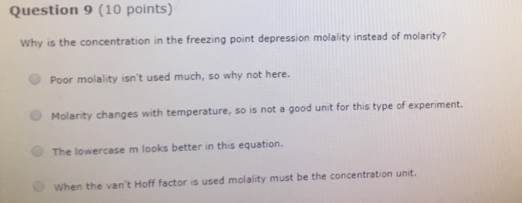# Problem: Question 9 (10 points) Why is the concentration in the freezing point depression molality instead of molarity? (i) Poor molality isn't used much, so why not here. (ii) Molarity changes with temperature, so is not a good unit for this type of experiment. (iii) The lowercase m looks better in this equation. (iv) when the van't Hoff factor is used molality must be the concentration unit.

###### FREE Expert Solution

(i) Poor molality isn't used much, so why not here. (False)

94% (449 ratings)###### Problem Details

Question 9 (10 points)

Why is the concentration in the freezing point depression molality instead of molarity?

(i) Poor molality isn't used much, so why not here.

(ii) Molarity changes with temperature, so is not a good unit for this type of experiment.

(iii) The lowercase m looks better in this equation.

(iv) when the van't Hoff factor is used molality must be the concentration unit.